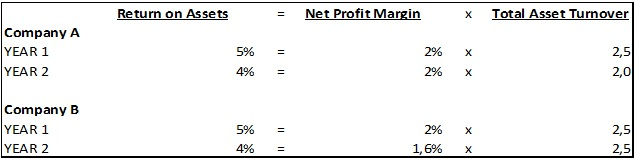DuPont Analysis as a Method of Measuring Company's Profitability

General information on DuPont analysis

The ability of an enterprise to earn profit is called profitability, and this is the main objective of the business. Company’s profitability measurement is an object of interest of its creditors, investors, managers. The most usual tool of this measurement is the profitability ratio analysis, performance of which means numerous business ratios calculation and interpretation in order to make conclusions on firm’s profit generation ability. DuPont analysis is an element of the profitability ratio analysis, which is often being applied to firm’s financial statement in order to measure its profitability through a few key ratios: return on assets, return on equity, net profit margin, total asset turnover and financial leverage.

Principles and interpretation of DuPont analysis

Return on assets, net profit margin, and total assets turnover are among the most commonly used ratios in the firm’s earning ability estimation process. Separately these ratios measure firm’s profitability and activity from different views. The DuPont company was the first to break the calculation of the return on assets ratio in two separate parts: calculation of the net profit margin and the total asset turnover. Doing this they developed their own formula, which demonstrated the correlation between these three ratios. This formula looks as follows:

Net Income Before Noncontrolling Interest and Nonrecurring Items ÷ Average Total Assets = Net Income Before Noncontrolling Interest and Nonrecurring Items ÷ Net Sales × Net Sales ÷ Average Total Assets

The main goal of such decomposition is to reveal the basic factors of efficiency. For example, slow asset turnover may witness the influence of firm’s bad asset management on the total return on assets, and thus notify the company’s management to make some decisions on improvement. In other words, DuPont return on assets can be described with the following formula:

Return on Assets (ROA) = Net Income ÷ Sales × Sales ÷ Total Assets

Take a look at the following example to understand the reasons for splitting the calculation of the return on assets into parts:This table shows the information about two companies with a similar declining trend of return on assets. It declined from 5% in year 1 to 4% in year 2 for both of them. However, key causes of the decline were different. Company A slowed down the turnover of its assets, and Company B reduced its net profit margin. Both processes have led to the same result.

As mentioned before, DuPont interpretation is also available for the return on equity formula, and it looks as follows:

Return on Equity (ROE) = Net Income ÷ Sales × Sales ÷ Total Equity

Same as with DuPont return on assets, this formula shows the processes that influence firm’s return on equity, and the efficiency of use of funds, invested in a firm by its owners. Another way to calculate DuPont return on equity is as follows:

Return on Equity (ROE) = Net Profit Margin × Total Asset Turnover × Equity Multiplier

In a situation, when two companies have the same return on equity ratio, investors should use the DuPont approach and deconstruct the calculation to details. Having higher net profit margin and better asset turnover is preferable, even if the equity multiplier is lower because this would indicate good management level of a company, and the capital structure is easily changeable.

More detailed formula for the DuPont return on equity calculation looks as follows:

Return on Equity (ROE) = Net Income ÷ Sales × Sales ÷ Assets × Assets ÷ Equity

Summary

DuPont analysis is a form of calculation of common business ratios, where profitability ratios, such as return on equity and return on assets are being interpreted through other ratios. This is being done to estimate the reasons of changes in profitability, measure the effect of company’s management on it and make appropriate business decisions.